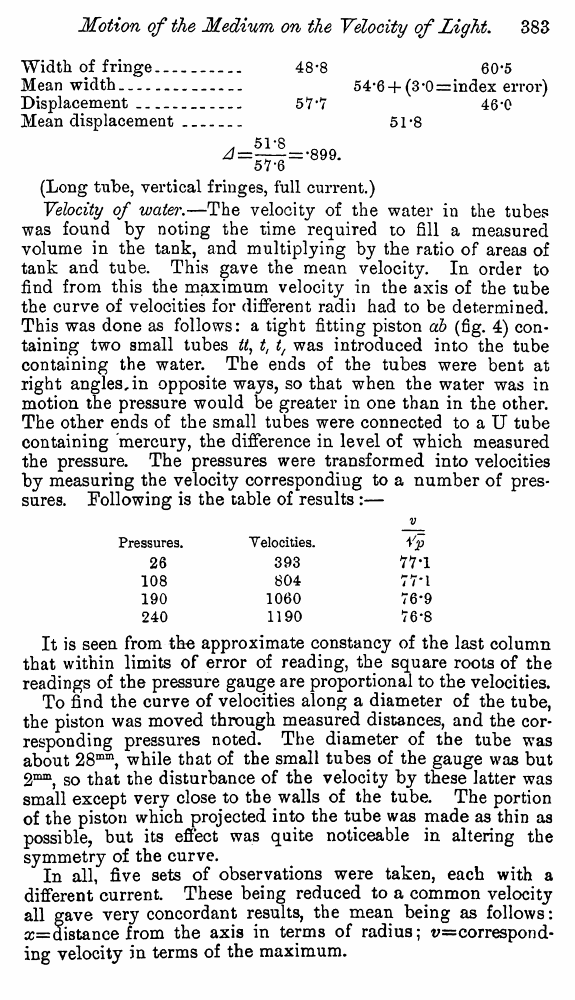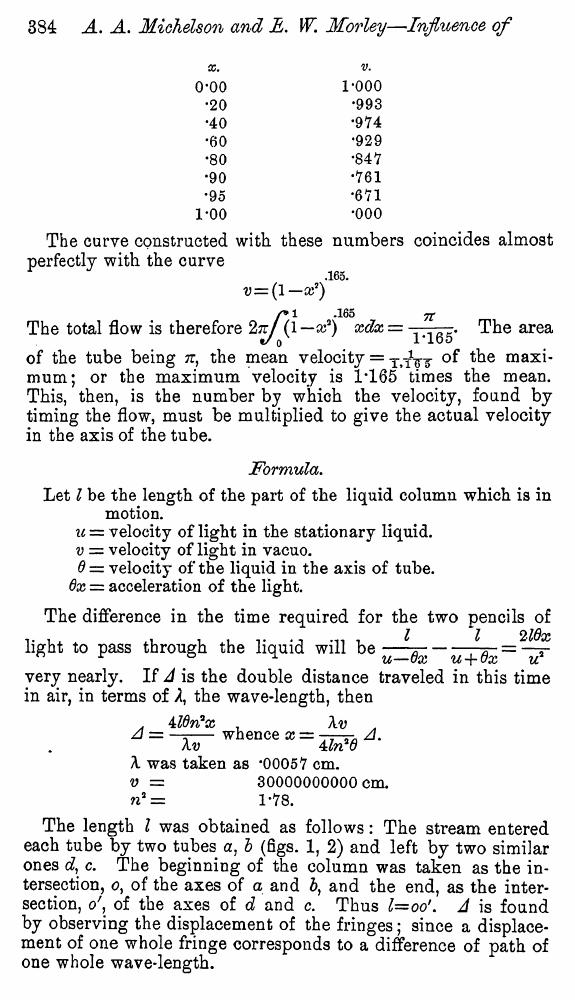# Michelson A.A., Morley E. Influence of Motion of the Medium on the velocity of Light. // American Journal of Science, Ser. 3, 1886, vol. 31

В начало   Другие форматы   <<<     Страница 383   >>>377  378  379  380  381  382  383 384  385  386

Width of fringe - _.

Mean width.......

Displacement_____

Mean displacement

48*8

60*5

54*6 +(3-0=index error) 46*0

51-8

(Long tube, vertical fringes, full current.)

Velocity of water.—The velocity of the water in the tubes was found by noting the time required to fill a measured volume in the tank, and multiplying by the ratio of areas of tank and tube. This gave the mean velocity. In order to find from this the maximum velocity in the axis of the tube the curve of velocities for different radii had to be determined. This was done as follows: a tight fitting piston db (fig. 4) containing two small tubes W, t, t, was introduced into the tube containing the water. The ends of the tubes were bent at right angles, in opposite ways, so that when the water was in motion the pressure would be greater in one than in the other. The other ends of the small tubes were connected to a U tube containing mercury, the difference in level of which measured the pressure. The pressures were transformed into velocities by measuring the velocity corresponding to a number of pressures. Following is the table of results :—

It is seen from the approximate constancy of the last column that within limits of error of reading, the square roots of the readings of the pressure gauge are proportional to the velocities.

To find the curve of velocities along a diameter of the tube, the piston was moved through measured distances, and the corresponding pressures noted. The diameter of the tube was about 28mm, while that of the small tubes of the gauge was but 2mm, so that the disturbance of the velocity by these latter was small except very close to the walls of the tube. The portion of the piston which projected into the tube was made as thin as possible, but its effect was quite noticeable in altering the symmetry of the curve.

In all, five sets of observations were taken, each with a different current. These being reduced to a common velocity all gave very concordant results, the mean being as follows: x= distance from the axis in terms of radius; v=correspond-ing velocity in terms of the maximum.

V

Pressures.

26

108

190

240

Velocities.

393

804

1060

1190

Vp

77*1

TV'l

76*9

76-8

 X. V. 00 1*000 20 *993 40 *974 60 •929 80 *847 90 *761 95 *671 00 *000

The curve constructed with, these numbers coincides almost perfectly with the curve

.165.

V = (l—X7)

r* 1 .165 ft

The total flow is therefore 2tu (l—a;3) xdx = . The area

of the tube being jt, the mean velocity = °? ^e mum; or the maximum velocity is 1*165 times the mean. This, then, is the number by which the velocity, found by timing the flow, must be multiplied to give the actual velocity in the axis of the tube.

Formula.

Let I be the length of the part of the liquid column which is in motion.

u = velocity of light in the stationary liquid. v = velocity of light in vacuo.

6 = velocity of the liquid in the axis of tube.

Ox = acceleration of the light.

The difference in the time required for the two pencils of

I 1/ 216x

light to pass through the liquid will be-tt;---t*-= —*~

U~~OX U “p 0%, u

very nearly. If A is the double distance traveled in this time in air, in terms of A, the wave-length, then

MOtfx . Xv .

whence x = ■A

Xv 4 ln*d

X was taken as *00057 cm. v = 30000000000 cm.

n2 = 1*78.

The length I was obtained as follows: The stream entered each tube by two tubes a, b (figs. 1, 2) and left by two similar ones e£, c. The beginning of the column was taken as the intersection, o, of the axes of a and 5, and the end, as the intersection, o', of the axes of d and c. Thus l=oo'. A is found by observing the displacement of the fringes; since a displacement of one whole fringe corresponds to a difference of path of one whole wave-length.